Affine Transformation

Brief Description

In many imaging systems, detected images are subject to geometric distortion introduced by perspective irregularities wherein the position of the camera(s) with respect to the scene alters the apparent dimensions of the scene geometry. Applying an affine transformation to a uniformly distorted image can correct for a range of perspective distortions by transforming the measurements from the ideal coordinates to those actually used. (For example, this is useful in satellite imaging where geometrically correct ground maps are desired.)
An affine transformation is an important class of linear 2-D geometric transformations which maps variables (e.g. pixel intensity values located at position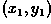in an input image) into new variables (e.g.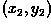in an output image) by applying a linear combination of translation, rotation, scaling and/or shearing (i.e. non-uniform scaling in some directions) operations.How It Works

In order to introduce the utility of the affine transformation, consider the image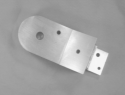wherein a machine part is shown lying in a fronto-parallel plane. The circular hole of the part is imaged as a circle, and the parallelism and perpendicularity of lines in the real world are preserved in the image plane. We might construct a model of this part using these primitives; however, such a description would be of little use in identifying the part from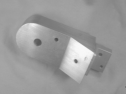Here the circle is imaged as an ellipse, and orthogonal world lines are not imaged as orthogonal lines.
This problem of perspective can be overcome if we construct a shape description which is invariant to perspective projection. Many interesting tasks within model based computer vision can be accomplished without recourse to Euclidean shape descriptions (i.e. those requiring absolute distances, angles and areas) and, instead, employ descriptions involving relative measurements (i.e. those which depend only upon the configuration's intrinsic geometric relations). These relative measurements can be determined directly from images. Figure 1 shows a hierarchy of planar transformations which are important to computer vision.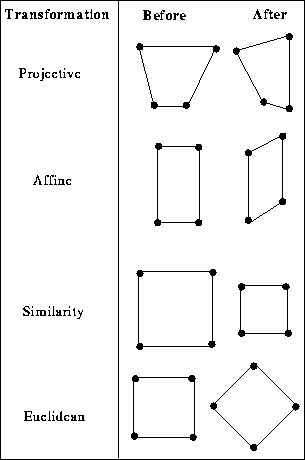Figure 1 Hierarchy of plane to plane transformation fromEuclidean (where only rotations and translations are allowed) to Projective (where a square can be transformed into any more general quadrilateral where no 3 points are collinear). Note that transformations lower in the table inherit the invariants of those above, but because they possess their own groups of definitive axioms as well, the converse is not true.

The transformation of the part face shown in the example image above is approximated by a planar affine transformation. (Compare this with the image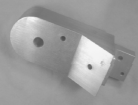where the distance to the part is not large compared with its depth and, therefore, parallel object lines begin to converge. Because the scaling varies with depth in this way, a description to the level of projective transformation is required.) An affine transformation is equivalent to the composed effects of translation, rotation, isotropic scaling and shear.
The general affine transformation is commonly written in homogeneous coordinates as shown below: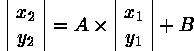By defining only the B matrix, this transformation can carry out pure translation: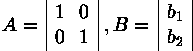Pure rotation uses the A matrix and is defined as: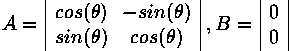Similarly, pure scaling is: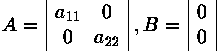(Note that several different affine transformations are often combined to produce a resultant transformation. The order in which the transformations occur is significant since a translation followed by a rotation is not necessarily equivalent to the converse.)
Since the general affine transformation is defined by 6 constants, it is possible to define this transformation by specifying the new output image locationsof any three input image coordinatepairs. (In practice, many more points are measured and a least squares method is used to find the best fitting transform.)Guidelines for Use

Most implementations of the affine operator allow the user to define a transformation by specifying to where 3 (or less) coordinate pairs from the input imagere-map in the output image. (It is often the case, as with the implementation used here, that the user is restricted to re-mapping corner coordinates of the input image to arbitrary new coordinates in the output image.) Once the transformation has been defined in this way, the re-mapping proceeds by calculating, for each output pixel location, the corresponding input coordinates. If that input point is outside of the image, then the output pixel is set to the background value. Otherwise, the value of (i) the input pixel itself, (ii) the neighbor nearest to the desired pixel position, or (iii) a bilinear interpolation of the neighboring four pixels is used.
We will illustrate the operation of the affine transformation by applying a series of special-case transformations (e.g. pure translation, pure rotation and pure scaling) and then some more general transformations involving combinations of these.
Starting with the 256×256 binary artificial image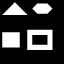we can apply a translation using the affine operator in order to obtain the imageIn order to perform this pure translation, we define a transformation by re-mapping a single point (e.g. the input image lower-left corner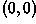) to a new position at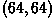.
A pure rotation requires re-mapping the position of two corners to new positions. If we specify that the lower-left corner moves to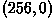and the lower-right corner moves to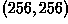, we obtainSimilarly, reflection can be achieved by swapping the coordinates of two opposite corners, as shown in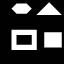Scaling can also be applied by re-mapping just two corners. For example, we can send the lower-left corner to, while pinning the upper-right corner down at, and thereby uniformly shrink the size of the image subject by a quarter, as shown in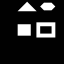Note that here we have also translated the image. Re-mapping any 2 points can introduce a combination of translation, rotation and scaling.
A general affine transformation is specified by re-mapping 3 points. If we re-map the input image so as to move the lower-left corner up toalong the 45 degree oblique axis, move the upper-right corner down by the same amount along this axis, and pin the lower-right corner in place, we obtain an image which shows some shearing effectsNotice how parallel lines remain parallel, but perpendicular corners are distorted.
Affine transformations are most commonly applied in the case where we have a detected image which has undergone some type of distortion. The geometrically correct version of the input image can be obtained from the affine transformation by re-sampling the input image such that the information (or intensity) at each pointis mapped to the correct positionin a corresponding output image.
One of the more interesting applications of this technique is in remote sensing. However, because most images are transformed before they are made available to the image processing community, we will demonstrate the affine transformation with the terrestrial image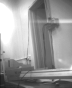which is a contrast-stretched (cutoff fraction = 0.9) version of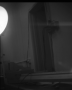We might want to transform this image so as to map the door frame back into a rectangle. We can do this by defining a transformation based on a re-mapping of the (i) upper-right corner to a position 30% lower along the y-axis, (ii) the lower-right corner to a position 10% lower along the x-axis, and (iii) pinning down the upper-left corner. The result is shown in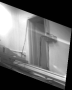Notice that we have defined a transformation which works well for objects at the depth of the door frame, but nearby objects have been distorted because the affine plane transformation cannot account for distortions at widely varying depths.
It is common for imagery to contain a number of perspective distortions. For example, the original image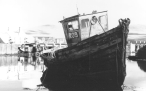shows both affine and projective type distortions due to the proximity of the camera with respect to the subject. After affine transformation, we obtain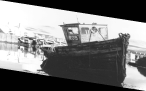Notice that the front face of the captain's house now has truly perpendicular angles where the vertical and horizontal members meet. However, the far background features have been distorted in the process and, furthermore, it was not possible to correct for the perspective distortion which makes the bow appear much larger than the hull,

Exercises

1. It is not always possible to accurately represent the distortion in an image using an affine transformation. In what sorts of imaging scenarios would you expect to find non-linearities in a scanning process and/or differences in along-scans vs across-scans?

2. Apply an affine transformation to the imagea) Experiment with different combinations of basic translation, rotation and scaling and then apply a transform which combines several of these operations. b) Rotate a translated version of the image and compare your result with the result of translating a rotated version of the image.

References

A. Jain Fundamentals of Digital Image Processing, Prentice-Hall, 1986, p 321.
B. Horn Robot Vision, MIT Press, 1986, pp 314 - 315.
D. Marr Vision, Freeman, 1982, p 185.
A. Zisserman Notes on Geometric and Invariance in Vision, British Machine Vision Association and Society for Pattern Recognition, 1992, Chap. 2.